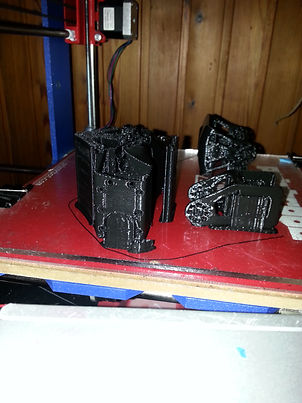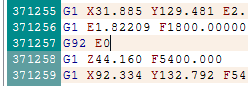We've all experienced it at some point. Your prints just randomly stopping, your extruder getting clogged mid way, or anything else, the result is the same: when you come back to your printer all you see is half a print.Step One:• Measure the height your print managed to achieve.

The height measured was 44.2mm, in this case I am using a layer height of .32mm with a first layer height of also .32mm.

• Subtract the first layer height from the total height, then divide the answer by your normal layer height

(44.2 - 0.32)/0.32=137.125

Of course, this value needs to be a whole number, and is a result of the tolerance of our measurement. this is why we did this calculation!

• round the answer down to the nearest number

in this case 137. (This is (+1) is the number of layers)

• multiply that by the layer height, add the first layer height

(137*0.32)+0.32=44.16. This is the height achieved, as far as the Gcode is concerned.

Step twoEdit your Gcode. In this case, I am using RepetierHost, but you may use any program to edit your code.• Search for the line of code indicating the Z-value. Add a Z before the calculated height

In my case, I need to search for Z44.16• click to select the end of the previous line of code• Then scroll up and, while pressing shift, click on the end of your start Gcode (all the descriptive code)• Step three